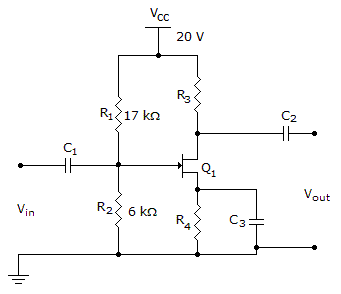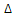# Electronics - Field Effect Transistors (FET)

### Exercise :: Field Effect Transistors (FET) - General Questions

26.

The transconductance curve of a JFET is a graph of:

 A. IS versus VDS B. IC versus VCE C. ID versus VGS D. ID × RDS

Explanation:

No answer description available for this question. Let us discuss.

27.

The common-source JFET amplifier has:

 A. a very high input impedance and a relatively low voltage gain B. a high input impedance and a very high voltage gain C. a high input impedance and a voltage gain less than 1 D. no voltage gain

Explanation:

No answer description available for this question. Let us discuss.

28.

Using voltage-divider biasing, what is the voltage at the gate VGS?A. 5.2 V B. 4.2 V C. 3.2 V D. 2.2 V

Explanation:

No answer description available for this question. Let us discuss.

29.

The overall input capacitance of a dual-gate D-MOSFET is lower because the devices are usually connected:

 A. in parallel B. with separate insulation C. with separate inputs D. in series

Explanation:

No answer description available for this question. Let us discuss.

30.

What is the transconductance of an FET whenID = 1 mA andVGS = 1 V?

 A. 1 kS B. 1 mS C. 1 kD. 1 m